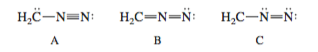Problem: Consider Lewis formulas A, B, and C:(a) Are A, B, and C constitutional isomers, or are they resonance contributors?

FREE Expert Solution
100% (341 ratings)
Problem Details

Consider Lewis formulas A, B, and C:

(a) Are A, B, and C constitutional isomers, or are they resonance contributors?What scientific concept do you need to know in order to solve this problem?

Our tutors have indicated that to solve this problem you will need to apply the Resonance Structures-1 concept. If you need more Resonance Structures-1 practice, you can also practice Resonance Structures-1 practice problems .

What is the difficulty of this problem?

Our tutors rated the difficulty of Consider Lewis formulas A, B, and C:(a) Are A, B, and C cons... as medium difficulty.

How long does this problem take to solve?

Our expert Organic tutor, Jonathan took 6 minutes to solve this problem. You can follow their steps in the video explanation above.

What professor is this problem relevant for?

Based on our data, we think this problem is relevant for Professor Hashim's class at UCF.

What textbook is this problem found in?

Our data indicates that this problem or a close variation was asked in . You can also practice practice problems .